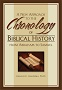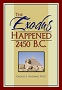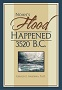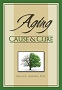Home

Resources
time charts
Topics
Overview
Biblical Chronology Dendrochronology Radiocarbon Dating     Mt. Sinai Jericho Ai The Exodus Noah's Ark Imhotep/Joseph Anti-Aging Vitamins
Correspondence

## How precise is radiocarbon dating?

A common misconception about radiocarbon dating is that it gives a precise date---3577 B.C., for example. In actual practice radiocarbon dating can only give a range of dates for a given sample---3650 to 3410 B.C., for example---the true date lying somewhere in that range. The precision of a radiocarbon date tells how narrow the range of dates is. There are two main factors which determine the precision of a radiocarbon date.

### 1. The precision of the measurement of the radiocarbon concentration in the sample.

Modern accelerator mass spectrometry (used for radiocarbon dating purposes to separate radiocarbon atoms from stable carbon atoms and count them) is quite precise. The technology involved is fascinating and impressive. Measurements can be made with a high degree of precision. For example, Dr. Aardsma submitted a sample from a reed mat known to be over 5,000 years old. The measurement, before calibration, came back with an error bar of +/- about 60 radiocarbon years. That is only 1.2% measurement uncertainty.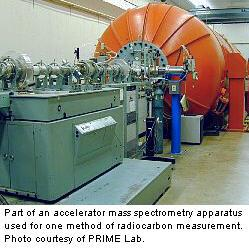It should be noted that these measurement uncertainties do not increase linearly as one goes back in time. In Accelerator Mass Spectrometry (AMS), for example, the number of radiocarbon atoms in a stream of atoms coming from the sample is counted. Thus there are statistical counting uncertainties proportional to the square root of the number of atoms counted. Older samples have a lower concentration of radiocarbon, but they can be (and often are) counted for longer periods of time to compensate for this lower concentration. By counting longer, the counting uncertainty in a radiocarbon measurement on a very old sample can be the same as that on a young sample.

### 2. The precision of the calibration process.

Once the radiocarbon concentration in a sample has been measured, the sample's age in "radiocarbon years" is determined mathematically. The radiocarbon age must then be calibrated to determine the sample's age in calendar years. Modern radiocarbon dating uses tree-ring chronologies to produce the calibration curve.

Because the radiocarbon to stable carbon ratio in the atmosphere has fluctuated over time, there are "wiggles" in the calibration curve. Thus it is possible in some instances for two samples from a few decades apart to have the same radiocarbon concentration today, and hence the same apparent radiocarbon age. This happens whenever there is a wiggle in the curve at the time the samples died. This, in fact, is the most significant factor contributing to loss of precision in radiocarbon dates today. However, this contribution is usually only a few decades.